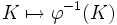# Fourth isomorphism theorem

## Contents

This article gives the statement, and possibly proof, of a basic fact in group theory.
View a complete list of basic facts in group theory
VIEW FACTS USING THIS: directly | directly or indirectly, upto two steps | directly or indirectly, upto three steps|
View a complete list of isomorphism theorems| Read a survey article about the isomorphism theorems

## Name

This result is termed the lattice isomorphism theorem, the fourth isomorphism theorem, and the correspondence theorem.

## Statement

### Statement with symbols

Let$G$ be a group and let$N$ be a Normal subgroup (?) of$G$. Then, we have a bijection:

Set of subgroups of$G$ containing$N$$\leftrightarrow$ Set of subgroups of$G/N$

If$\varphi:G \to G/N$ is the quotient map, then this bijection is given by:$H \mapsto \varphi(H)$

in the forward direction, and:$K \mapsto \varphi^{-1}(K)$

in the reverse direction. Moreover:

1. Under the bijection, normality is preserved. In other words, a subgroup containing$N$ is normal if and only if its image under$\varphi$ is normal.
2. The bijection is an isomorphism between the lattice of subgroups of$G$ containing$N$, and the lattice of subgroups of$G/N$. In other words, the bijection preserves partial order:$A \le B$ if and only if$\varphi(A) \le \varphi(B)$. It also preserves intersections and joins.
3. The bijection preserves index. If$A,B$ are subgroups of$G$ containing$N$, with$A \le B$, then$[B:A] = [\varphi(B):\varphi(A)]$.